Courses

# Irrational Numbers - Number System, Class 9 Math

## 25 Questions MCQ Test Mathematics (Maths) Class 9 | Irrational Numbers - Number System, Class 9 Math

Description
This mock test of Irrational Numbers - Number System, Class 9 Math for Class 9 helps you for every Class 9 entrance exam. This contains 25 Multiple Choice Questions for Class 9 Irrational Numbers - Number System, Class 9 Math (mcq) to study with solutions a complete question bank. The solved questions answers in this Irrational Numbers - Number System, Class 9 Math quiz give you a good mix of easy questions and tough questions. Class 9 students definitely take this Irrational Numbers - Number System, Class 9 Math exercise for a better result in the exam. You can find other Irrational Numbers - Number System, Class 9 Math extra questions, long questions & short questions for Class 9 on EduRev as well by searching above.
QUESTION: 1

### A number is irrational if and only if its decimal representation is:

Solution:

A non-terminating, non-repeating decimal is a decimal number that continues endlessly, with no group of digits repeating endlessly. Decimals of this type cannot be represented as fractions, and as a result, are irrational numbers. Pi is a non-terminating, non-repeating decimal.

QUESTION: 2

### The product or quotient of a non-zero rational number with an irrational number is:

Solution:

The quotient of a non zero rational number with an irrational number will be irrational.

QUESTION: 3

### A rational number between √3 and √5 is

Solution: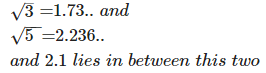QUESTION: 4

Which of the following statement about real numbers is false ?

Solution:
QUESTION: 5

(√12 + √10 - √2) is

Solution:
QUESTION: 6

The value obtained on simplifying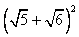Solution:
QUESTION: 7

The decimal expansion 0.080080008000080000080000008……. is a

Solution:
QUESTION: 8

Sam bought 2 7⁄5 kg of flour in one week and 3 4⁄15 kg of flour in second week. The flour Sam bough altogether is

Solution:
QUESTION: 9

An irrational number between 5/7 and 7/9 is :

Solution:
QUESTION: 10

Identify the irrational number among these.

Solution:
QUESTION: 11

On simplifying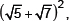we get

Solution:
QUESTION: 12

The product of two numbers is -20/9. If one of the numbers is 4, find the other.

Solution:
QUESTION: 13

Which of the following is an irrational number ?

Solution:
QUESTION: 14

Insert one rational number between 2 and 3.​

Solution:

A rational number between 2 and 3 =  2 + 3  / 2 = 2.5

An irrational number between 2 and 3 is 5 .

QUESTION: 15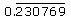= _______.

Solution:
QUESTION: 16

The ratio of the circumference of a circle to the diameter of the circle is.

Solution:

Circles are all similar, and "the circumference divided by the diameter" produces the same value regardless of their radius. This value is the ratio of the circumference of a circle to its diameter and is called π (Pi). This constant appears in the calculation of the area of a circle and is a type of an irrational number known as a transcendental number that can be expressed neither by a fraction nor by any radical sign such as a square root, nor their combination.

QUESTION: 17

Of the given numbers
(i) √27
(ii) √256
(iii) 0.3796
(iv) 7.478478…
(v) 1.101001000100001…

Solution:
QUESTION: 18

Which among these is the approximate value of π?

Solution:

The number π  is a mathematical constant. Originally defined as the ratio of a circle's circumference to its diameter, it now has various equivalent definitions and appears in many formulas in all areas of mathematics and physics. It is approximately equal to 3.14159. It has been represented by the Greek letter "π" since the mid-18th century, though it is also sometimes spelled out as "pi". It is also called Archimedes' constant.

QUESTION: 19

The decimal expansion 0.080080008000080000080000008….. is a

Solution:

The decimal expansion 0.080080008000080000080000008….. is a. Non-terminating, non-recurring. Non-terminating, recurring.

QUESTION: 20

How many rational numbers can you find between 5 and 6?​

Solution:

Infinitely many. You can say 5.1 is a rational number lying between 5 and 6. 5.01, 5.001, 5.0001, 5.00001 and so forth.

QUESTION: 21

Given that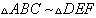∠A = 50° ∠C = 35° ∠E = ?

Solution:
QUESTION: 22

Which of the following statements is false?

Solution:
QUESTION: 23

Convert 7/25 in the form of decimals,

Solution: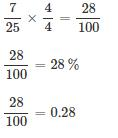QUESTION: 24

From the choices given below mark the co-prime numbers

Solution:
QUESTION: 25

Which of the following statement is true?

Solution:

The real numbers consist of both rational and irrational numbers. so the other three options are not true as the first one is not true because not only every real number is a rational number but the real number is rational and irrational both. same goes for the second one and the last option is not true because one half and the other half can be irrational or rational both.